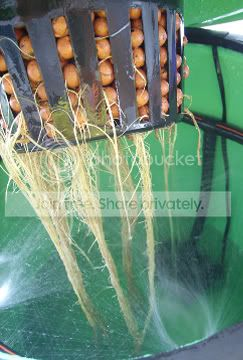# Describing Concentration in Hydroponics

Most amateur growers, both hobby and commercial, who do not have a chemical knowledge background are most of the time stunned by the amount of chemistry involved in hydroponic cultivation. One of the things that proves to be the most difficult for this new comers is the understanding and description of nutrient concentrations.

Concentration, to start, is just a way of expressing the amount of something inside something else. In hydroponics, we are interested in expressing the amount of nutrients per amount of nutrient solution. To do this, we use several tools available in chemistry.

In general, concentrations in hydroponics are either expressed as moles of nutrient per liter of solution or as milligrams of nutrient per liter of solutions. The first unit is called molarity while the second unit is generally referred to as ppm (parts per million). This means that a solution containing 12 ppm of Nitrogen contains 12mg of nitrogen per liter of solution and a solution containing nitrogen in a 0.001M concentrations contains 0.001 moles of nitrogen per liter of solution. The first form of concentration (ppm) is often used in hydroponics while the second (M) is used in hydroponics only in research papers and such.

In practice, the use of concentrations in ppm makes preparation a little bit easier as the leap from concentration to mass becomes easier if the unit of measurement inside the unit belongs to mass. Since the ppm unit can be translated as (mg/L) going to the mass of salt required is easier than with molarity (although not by much).

Another advantage that we come across when using ppm as a main unit of concentration measurement in hydroponics is that the numbers are likable. We, humans, tend to like numbers between 0 and 1000, so concentrations of 120 ppm, 250 ppm and such, seem much easier to grasp than their equivalents in molarity which would be 0.0012 M, 0.00023 M or the like.

In an article to be written soon, I intend to describe how to do the leap from this concentration measurements onto the mass of salt that is needed to weight in order to achieve the desired concentration inside a hydroponic solution. So stay tuned to know a little bit more about the science of hydroponic nutrient solution making !Subscribe Today!